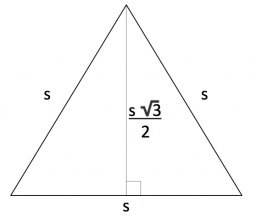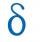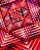# Infinite sum of areas

Above the height of the equilateral triangle ABC is constructed an equilateral triangle A1, B1, C1, of the height of the equilateral triangle built A2, B2, C2, and so on. The procedure is repeated continuously. What is the total sum of the areas of all triangles if the ABC triangle has a length? And?

Correct result:

s =  1.732 a2

#### Solution:

$S=\sqrt{ 3 }/4 \ a^2 \ \\ a_{1}=\sqrt{ 3 }/2 \ a \ \\ \ \\ S_{1}=\sqrt{ 3 }/4 \ a_{1}^2=\sqrt{ 3 }/4 \cdot \ ( \sqrt{ 3 }/2 \ a)^2 \ \\ S_{1}=3/4 \ S \ \\ \ \\ S_{2}=3/4 \ S_{1} \ \\ ... \ \\ \ \\ q=S_{2}/S_{1}=S_{1}/S=.. \ \\ q=\dfrac{ 3 }{ 4 }=0.75 \ \\ q<1 \ \\ \ \\ s=\dfrac{ \sqrt{ 3 }/4 }{ 1-q }=\dfrac{ \sqrt{ 3 }/4 }{ 1-0.75 }=\sqrt{ 3 }=1.732 \ \text{a}^2$Our examples were largely sent or created by pupils and students themselves. Therefore, we would be pleased if you could send us any errors you found, spelling mistakes, or rephasing the example. Thank you!

Please write to us with your comment on the math problem or ask something. Thank you for helping each other - students, teachers, parents, and problem authors.Tips to related online calculators
Need help calculate sum, simplify or multiply fractions? Try our fraction calculator.
Pythagorean theorem is the base for the right triangle calculator.

#### You need to know the following knowledge to solve this word math problem:

We encourage you to watch this tutorial video on this math problem:

## Next similar math problems:

• Recursion squaresIn the square ABCD is inscribed a square so that its vertices lie at the centers of the sides of the square ABCD.The procedure of inscribing square is repeated this way. Side length of square ABCD is a = 22 cm. Calculate: a) the sum of perimeters of all s
• Series and sequencesFind a fraction equivalent to the recurring decimal? 0.435643564356
• Decimal to fractionWrite decimal number 8.638333333 as a fraction A/B in the basic form. Given decimal has infinite repeating figures.
• Sum of seriesDetermine the 6-th member and the sum of a geometric series: 5-4/1+16/5-64/25+256/125-1024/625+....
• Free space in the gardenThe grandfather's free space in the garden was in the shape of a rectangular triangle with 5 meters and 12 meters in length. He decided to divide it into two parts and the height of the hypotenuse. For the smaller part creates a rock garden, for the large
• CalculateCalculate the length of a side of the equilateral triangle with an area of 50cm2.
• Euclid2In right triangle ABC with right angle at C is given side a=27 and height v=12. Calculate the perimeter of the triangle.
• RT triangle and heightCalculate the remaining sides of the right triangle if we know side b = 4 cm long and height to side c h = 2.4 cm.
• ABS CNCalculate the absolute value of complex number -15-29i.
• Triangle ABCIn a triangle ABC with the side BC of length 2 cm The middle point of AB. Points L and M split AC side into three equal lines. KLM is isosceles triangle with a right angle at the point K. Determine the lengths of the sides AB, AC triangle ABC.
• Vector 7Given vector OA(12,16) and vector OB(4,1). Find vector AB and vector |A|.
• GP membersThe geometric sequence has 10 members. The last two members are 2 and -1. Which member is -1/16?
• Geometric progression 2There is geometric sequence with a1=5.7 and quotient q=-2.5. Calculate a17.
• Six termsFind the first six terms of the sequence a1 = -3, an = 2 * an-1
• Difference APCalculate the difference of arithmetic progression if the sum of its first 19 members Sn = 8075 and the first member is a1 = 20
• CoefficientDetermine the coefficient of this sequence: 7.2; 2.4; 0.8
• Five elementThe geometric sequence is given by quotient q = 1/2 and the sum of the first six members S6 = 63. Find the fifth element a5.